# Alternating Current

## (1) Introduction

• We have already discussed about direct current (DC) which is produced by the voltage source whose pole does not change their polarity with time
• Hence direction of flow of direct current does not changes with time
• Alternating current on the other hand is produced by voltage source whose terminal polarity keeps alternating with time i.e. terminal which was positive at one instant of time becomes negative some time later and vice -versa
• Due to changing polarity of voltage source direction of flow of current also keep changing
• In this chapter we would learn how voltage and current changing with time are related to each other in various circuits with components namely resistors, capacitor and inductor.

## (2) Alternating current and Alternating EMF

• An alternating current is one whose magnitude changes sinusoidal with time .Thus alternating current is given by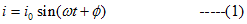Where
i0=current amplitude or peak value of alternating current
If T is the time period of alternating current and f is the frequency, then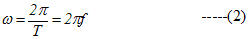Where ω is called angular frequency of A.C and φ is known as phase constant
• Instead of sine function AC can also be represented by cosine function and both representation leads to same results. We will discuss circuits with sine representation of A.C
• Figure below shows the variation of A.C with time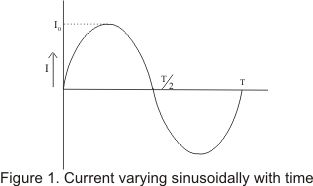• Complete set of variations of the current in one time period T is called cycle
• The emf or voltage whose magnitude changes sinusoidal with time is known as alternating emf and is represented by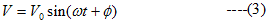where V0 is the peak value of alternating current.

## (3) Average or mean current

• When an alternating current passed through a moving coil galvanometer it shows no deflection ,this is because for one complete cycle mean value of alternating current is zero as AC flows in one direction during one half cycle and in opposite direction during another half cycle.
• But mean value of A.C is finite over half cycle.
• So, mean or average value of AC is defined either for positive half cycle or for negative half cycle
• So,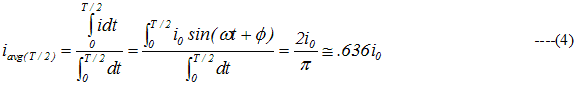• From equation (4),we see that the average value of A.C during the half cycle is .636 times or 63.6% of its peak value
• Similarly we can show that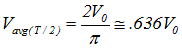• During next half cycle mean value of ac will be equal in magnitude but opposite in direction.
• Always remember that mean value of AC over a complete cycle is zero and is defined over a half cycle of AC.

Note to our visitors :-

Thanks for visiting our website.